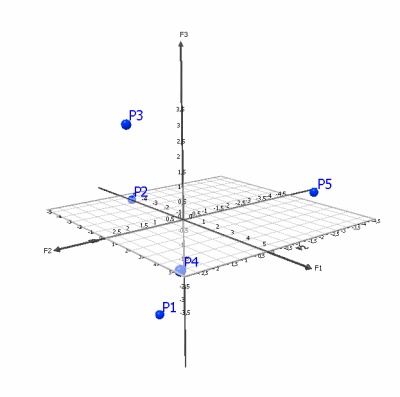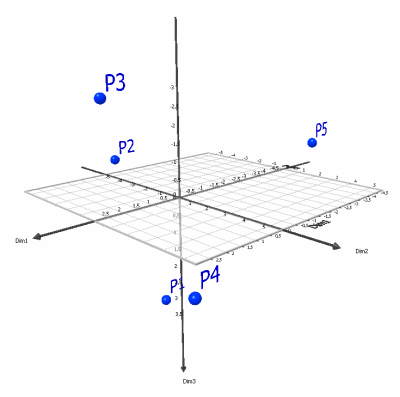# Principal Coordinate Analysis in Excel tutorial

2017-10-23

This tutorial will help you set up and interpretPrincipal Coordinate Analysis in Excel using the XLSTAT software.
Not sure if this is the right multivariate data analysis tool you need? Check out this guide.

## Principal Coordinate Analysis

Principal Coordinate Analysis (PCoA) is a method to represent on a 2 or 3 dimensional chart objects described by a square matrix containing resemblance indices between these objects.

This method is due to Gower (1966). It is sometimes called metric MDS (MDS: Mutidimensional scaling) as opposed to the MDS (or non-metric MDS). Both methods have the same objective and produce similar results if the similarity matrix of square distances are metric and if the dimensionality is sufficient.

## Dataset to run a Principal Coordinate Analysis

An Excel sheet with both the data and the results can be downloaded by clicking on the button below:

The data correspond to a survey performed over 10 testers which have been asked to rate (the score ranges from 1 to 5) five chocolate bars, where only the product P1 is already available on the market.

These data are also used in the tutorial on MDS. We will take advantage of that to compare the methods.

In the tutorial on the MDS, we show how to obtain, from these data, a matrix of Euclidean distances. We use here the table of Euclidean distances obtained before the MDS.

Our aim is to show how the products position themselves on a map, given the opinion of the testers.

A proximity matrix is needed to perform a MDS analysis, but here we have a individuals x products table. Therefore we need first to compute the dissimilarities between products, which can be done by using the Similarity / Dissimilarity matrix tool of XLSTAT.

## Setting up a Principal Coordinate Analysis

Once XLSTAT is activated, select the XLSTAT / Analyzing Data / Principal Coordinate Analysis.The dialog box appears. You can then select the data on the Excel sheet and choose the appropriate options as shown below.

The options of the Options tab are left unchanged as there is no risk of having negative eigenvalues in the case of a matrix with euclidean distances.Click on OK when the settings are completed.

## Interpreting the results of a Principal Coordinate Analysis

The following chart is created using the principal coordinates and allows to visualize the proximities between products in 2 dimensions.The table of squared cosines displayed in the report allows to avoid an error of interpretation: we see that the cosines for P1 and P3 are very low for both F1 and F2, indicating that these points are away from the F1/F2 plane. Looking at the table of coordinates we find that P1 and P3 which seem to be close on the F1/F2 map, are in reality far apart. The 3-dimensional chart produced with XLSTAT-3DPlot allows a better visualization.It is interesting to compare the outputs of the PCoA to those of the MDS. If the 3-dimensional representation is almost identical, it is quite different for what concerns the 2 dimensional map. This is explained by the difference of methods and of criteria that are optimized. To minimize the stress, MDS allows a deformation, while PCoA (as PCA) only allows rotations and projections: with PCA and PCoA, the 2D view is a projection of the 3D view on a 2D space that keeps as much variance as possible. With MDS, the 2D view is the distortion of the 3D configuration such that the distances between objects are respected as much as possible. The two methods are quite different although they share the same goal. Use MDS if just want a map where the distances and their ranking is respected. Use PCoA if you want a map that does not distort the "true" configuration and that simply rotates/projects it. To avoid wrong interpretations, using the squared cosines, PCoA gives you a way to check if points are truly close when they look alike. The solution of PCoA is unique, but MDS might have several local minimums.Note: A non-standardized (using the covariance matrix) PCA run on the 3-dimensional MDS configuration leads to a representation in 2 dimensions very similar to that obtained after the PCoA.

#### Contact our technical support team: support@xlstat.com

https://cdn.desk.com/
false
desk